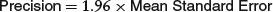APPENDIX C

Variance Reduction Techniques

As we saw in Chapter 12, one of the many uses of Monte Carlo simulation by financial analysts is to place a value on financial derivatives. You now know that you can sharpen the point estimate of your derivative’s value by using the brute force method of increasing the number of trials run during a simulation. However, there are also other relatively simple changes you can make to a model that provide dramatic increases in precision for a given number of simulation trials. Such changes made to a model are called variance-reduction techniques. This appendix, based on Charnes (2002), shows how the variance reduction techniques of antithetic variates (AV) and control variates (CV) can be used to sharpen the precision of your estimate of the value of an Asian call option.

The best point estimate of the value of a derivative is usually the mean, or arithmetic average, of the derivative’s discounted payoff taken over all trials of the simulation. One measure of the sharpness of the point estimate of the mean is Mean Standard Error, defined as

(C.1)The precision of the mean as a point estimate is often defined as the half-width of a 95 percent confidence interval, which is calculated as

(C.2)Lower values of Precision in Equation (C.2) correspond ...

Get Financial Modeling with Crystal Ball and Excel, + Website, 2nd Edition now with the O’Reilly learning platform.

O’Reilly members experience live online training, plus books, videos, and digital content from nearly 200 publishers.